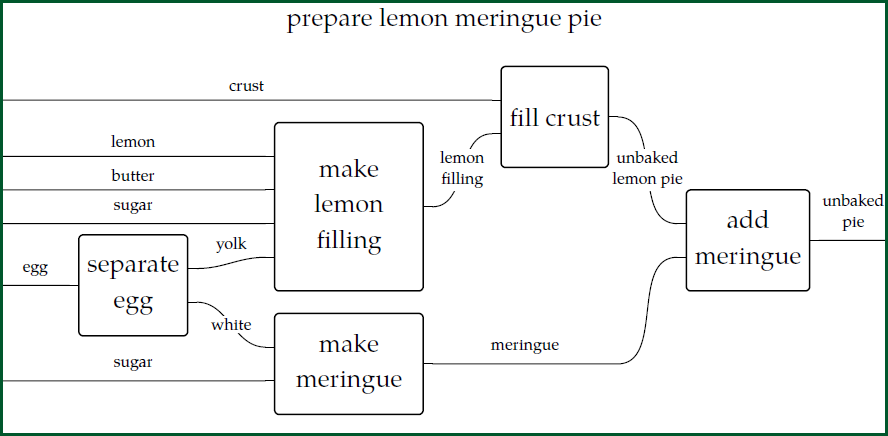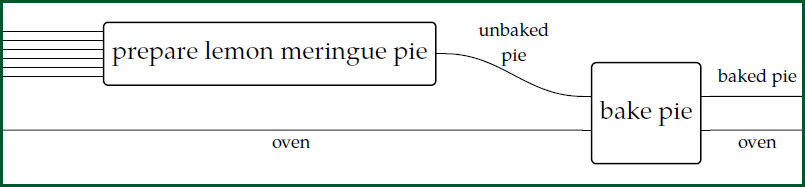Hi, I'm back from Leiden! I'm ready to rock and roll! Are you ready?

I could spend all day talking about the workshop on [applied category theory](https://johncarlosbaez.wordpress.com/2017/09/12/act-2018/) and what we did there. We figured out the math of how cells "couple" chemical reactions, we learned an amazing connection between ["lenses"](https://www.cis.upenn.edu/~bcpierce/papers/lenses-etapsslides.pdf) in bidirectional programming and [supervised learning](https://arxiv.org/abs/1711.10455) in artificial intelligence, we launched [a new journal](https://johncarlosbaez.wordpress.com/2018/05/06/compositionality/)... and much, much more. My brain is still recovering. But right now it's time to teach you some cool stuff!

Chapter 2 is about "resource theories". Resource theories help us answer questions like this:

1. Given what I have, _is it possible_ to get what I want?
2. Given what I have, _how much will it cost_ to get what I want?
3. Given what I have, _how long will it take_ to get what I want?
4. Given what I have, _what is the set of ways_ to get what I want?

In each of these questions we are given some "inputs" \$$x\$$ and we want some "outputs" \$$y\$$. In the first question we want a yes-or-no answer: can we get from \$$x\$$ to \$$y\$$. In the second and third questions we want a _number_ for an answer. In the fourth question we want a _set_ for an answer. We'll eventually get a unified framework for answering questions of all these kinds.

For example, we could be trying to make a lemon meringue pie. Have you ever made one? Not me! But here's a way to make one... at least before you put it into the oven:This kind of picture often called a "string diagram". Fong and Spivak call it a "wiring diagram", so we'll do that.

We can use this wiring diagram in many ways. The simplest way is to answer the first question in our list! _Yes, it is possible_ to make an unbaked lemon meringue pie if you have a crust, a lemon, some butter, some sugar, and egg, and some more sugar.

(Of course you'll also need some kitchen tools. These could be included in a more detailed string diagram. For now I'm trying to simplify things. That's also why I'm not saying _how much_ butter and sugar we need.)

Another thing we can do with this wiring diagram is connect it to _another_ wiring diagram and build a bigger wiring diagram, like this:Now we are baking our pie! For this we need an oven.

If all we care about is the first question in our list - _is it possible_ to go from some inputs to some outputs - wiring diagrams are overkill. For this question, we could summarize our first wiring diagram as an inequality:

$$\text{unbaked pie} \le \text{crust} + \text{lemon} + \text{butter} + \text{sugar} + \text{egg} + \text{sugar}$$

where we use \$$x \le y\$$ to mean "we can get \$$x\$$ from \$$y\$$". Similarly, we could summarize the baking process by

$$\text{baked pie} \le \text{unbaked pie} + \text{oven}$$

We can manipulate these inequalities in familiar ways to get

$$\text{baked pie} \le \text{crust} + \text{lemon} + \text{butter} + \text{sugar} + \text{egg} + \text{sugar} + \text{oven}$$

But what are these "familiar ways", exactly?

For starters, we are using the rules for a **[preorder](https://en.wikipedia.org/wiki/Preorder)**, which say that

$$x \le x$$

and

$$x \le y \textrm{ and } y \le z \textrm{ imply } x \le z .$$

That's one reason we went through [Chapter 1](http://www.azimuthproject.org/azimuth/show/Applied+Category+Theory#Chapter): to understand this stuff, you need to understand posets! But we are also using some rules about the symbol \$$+\$$. We're not doing ordinary addition of numbers here. So, what does this \$$+\$$ symbol really mean? More precisely: _what rules does should it obey?_

This question is one of the big topics of Chapter 2. If you want to peek ahead at the answer, read Section 2.2 of [our textbook](http://math.mit.edu/~dspivak/teaching/sp18/7Sketches.pdf).

But first I need to say more about why resource theories are important. We do not really need them to make lemon meringue pies! But we do need them to deeply understand chemistry, thermodynamics, operations research, project management, information theory and the study of quantum entanglement... and how these subjects fit together! So, I want to say a bit about that.

If you want to learn more about resource theories, besides our textbook and my lectures I recommend these papers:

* Bob Coecke, Tobias Fritz and Robert W. Spekkens, [A mathematical theory of resources](https://arxiv.org/abs/1409.5531).

* Tobias Fritz, [Resource convertibility and ordered commutative monoids](https://arxiv.org/abs/1504.03661).

The first is more elementary, so start there! Luckily [Tobias Fritz](https://forum.azimuthproject.org/discussion/2035/introduction-tobias-fritz/p1) is my friend, and he's attending this course - so once we get going, we can ask him some questions!

**[To read other lectures go here.](http://www.azimuthproject.org/azimuth/show/Applied+Category+Theory#Chapter_2)**